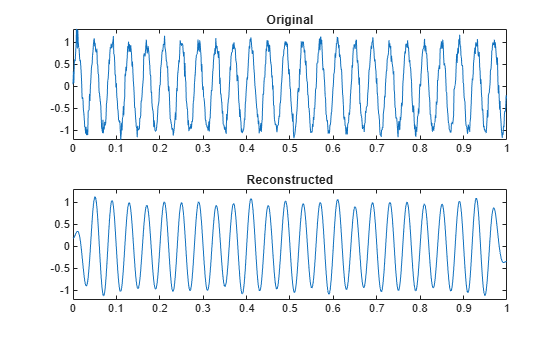# idct

Inverse discrete cosine transform

## Syntax

``x = idct(y)``
``x = idct(y,n)``
``x = idct(y,n,dim)``
``y = idct(___,'Type',dcttype)``

## Description

example

````x = idct(y)` returns the inverse discrete cosine transform of input array `y`. The output `x` has the same size as `y`. If `y` has more than one dimension, then `idct` operates along the first array dimension with size greater than 1.```
````x = idct(y,n)` zero-pads or truncates the relevant dimension of `y` to length `n` before transforming.```
````x = idct(y,n,dim)` computes the transform along dimension `dim`. To input a dimension and use the default value of `n`, specify the second argument as empty, `[]`.```

example

````y = idct(___,'Type',dcttype)` specifies the type of inverse discrete cosine transform to compute. See Inverse Discrete Cosine Transform for details. This option can be combined with any of the previous syntaxes.```

## Examples

collapse all

Generate a signal that consists of a 25 Hz sinusoid sampled at 1000 Hz for 1 second. The sinusoid is embedded in white Gaussian noise with variance 0.01.

```rng('default') Fs = 1000; t = 0:1/Fs:1-1/Fs; x = sin(2*pi*25*t) + randn(size(t))/10;```

Compute the discrete cosine transform of the sequence. Determine how many of the 1000 DCT coefficients are significant. Choose 1 as the threshold for significance.

```y = dct(x); sigcoeff = abs(y) >= 1; howmany = sum(sigcoeff)```
```howmany = 17 ```

Reconstruct the signal using only the significant components.

```y(~sigcoeff) = 0; z = idct(y);```

Plot the original and reconstructed signals.

```subplot(2,1,1) plot(t,x) yl = ylim; title('Original') subplot(2,1,2) plot(t,z) ylim(yl) title('Reconstructed')```Verify that the different variants of the discrete cosine transform are orthogonal, using a random signal as a benchmark.

Start by generating the signal.

`s = randn(1000,1);`

Verify that DCT-1 and DCT-4 are their own inverses.

```dct1 = dct(s,'Type',1); idt1 = idct(s,'Type',1); max(abs(dct1-idt1))```
```ans = 1.3323e-15 ```
```dct4 = dct(s,'Type',4); idt4 = idct(s,'Type',4); max(abs(dct4-idt4))```
```ans = 1.3323e-15 ```

Verify that DCT-2 and DCT-3 are inverses of each other.

```dct2 = dct(s,'Type',2); idt2 = idct(s,'Type',3); max(abs(dct2-idt2))```
```ans = 4.4409e-16 ```
```dct3 = dct(s,'Type',3); idt3 = idct(s,'Type',2); max(abs(dct3-idt3))```
```ans = 1.1102e-15 ```

## Input Arguments

collapse all

Input discrete cosine transform, specified as a real-valued or complex-valued vector, matrix, N-D array, or `gpuArray` object.

See Run MATLAB Functions on a GPU (Parallel Computing Toolbox) and GPU Support by Release (Parallel Computing Toolbox) for details on `gpuArray` (Parallel Computing Toolbox) objects.

Example: `dct(sin(2*pi*(0:255)/4))` specifies the discrete cosine transform of a sinusoid.

Example: `dct(sin(2*pi*[0.1;0.3]*(0:39))')` specifies the discrete cosine transform of a two-channel sinusoid.

Data Types: `single` | `double`
Complex Number Support: Yes

Inverse transform length, specified as a positive integer scalar.

Data Types: `single` | `double`

Dimension to operate along, specified as a positive integer scalar.

Data Types: `single` | `double`

Inverse discrete cosine transform type, specified as a positive integer scalar from 1 to 4.

Data Types: `single` | `double`

## Output Arguments

collapse all

Inverse discrete cosine transform, returned as a real-valued or complex-valued vector, matrix, N-D array, or `gpuArray` object.

collapse all

### Inverse Discrete Cosine Transform

The inverse discrete cosine transform reconstructs a sequence from its discrete cosine transform (DCT) coefficients. The `idct` function is the inverse of the `dct` function.

The DCT has four standard variants. For a transformed signal y of length N, and with δkℓ the Kronecker delta, the inverses are defined by:

• Inverse of DCT-1:

`$x\left(n\right)=\sqrt{\frac{2}{N-1}}\sum _{k=1}^{N}y\left(k\right)\frac{1}{\sqrt{1+{\delta }_{k1}+{\delta }_{kN}}}\frac{1}{\sqrt{1+{\delta }_{n1}+{\delta }_{nN}}}\mathrm{cos}\left(\frac{\pi }{N-1}\left(k-1\right)\left(n-1\right)\right)$`

• Inverse of DCT-2:

`$x\left(n\right)=\sqrt{\frac{2}{N}}\sum _{k=1}^{N}y\left(k\right)\frac{1}{\sqrt{1+{\delta }_{k1}}}\mathrm{cos}\left(\frac{\pi }{2N}\left(k-1\right)\left(2n-1\right)\right)$`

• Inverse of DCT-3:

`$x\left(n\right)=\sqrt{\frac{2}{N}}\sum _{k=1}^{N}y\left(k\right)\frac{1}{\sqrt{1+{\delta }_{n1}}}\mathrm{cos}\left(\frac{\pi }{2N}\left(2k-1\right)\left(n-1\right)\right)$`

• Inverse of DCT-4:

`$x\left(n\right)=\sqrt{\frac{2}{N}}\sum _{k=1}^{N}y\left(k\right)\mathrm{cos}\left(\frac{\pi }{4N}\left(2k-1\right)\left(2n-1\right)\right)$`

The series are indexed from n = 1 and k = 1 instead of the usual n = 0 and k = 0, because MATLAB® vectors run from 1 to N instead of from 0 to N – 1.

All variants of the DCT are unitary (or, equivalently, orthogonal): To find the forward transforms, switch k and n in each definition. DCT-1 and DCT-4 are their own inverses. DCT-2 and DCT-3 are inverses of each other.

 Jain, A. K. Fundamentals of Digital Image Processing. Englewood Cliffs, NJ: Prentice-Hall, 1989.

 Oppenheim, Alan V., Ronald W. Schafer, and John R. Buck. Discrete-Time Signal Processing. 2nd Ed. Upper Saddle River, NJ: Prentice Hall, 1999.

 Pennebaker, W. B., and J. L. Mitchell. JPEG Still Image Data Compression Standard. New York: Van Nostrand Reinhold, 1993.